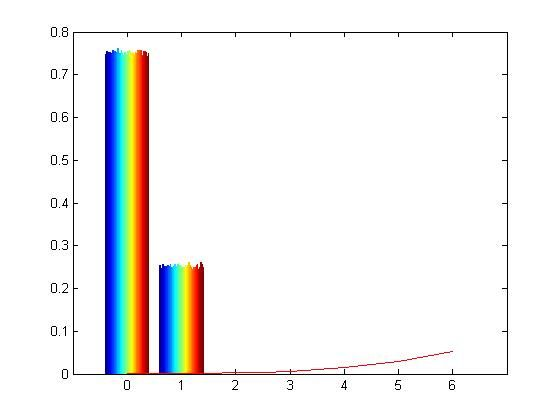# binomial simulation on matlab

#### Jeonsah

##### New member
Well if anyone has experience with matlab and probability, help would be greatly appreciated. The question is:
Binomial: If y = sumation from i =1 to n of a random variable Xi and Xi are Bernoulli(p) RV's(equal to 1 with probability p and equal to 0 with probability of 1-p), then Y has the Binomial pmf. For n = 50and p = 0.2, generate 10,000Rv's Y and plot the histogram, overlaying the Binomial pmf. Also overlay a N(np,np(1-p)) distribution to show that the Binomial pmf may e approximated with a Gaussian pdf.

Here is my code:
p = .2;
pn = (1-p);
mean = 50*p;
variance = mean*pn;
stddev = sqrt(variance);
x = rand([10000 50]);
y = zeros(size(x));

y(x>=0 & x < 0.2) = 1;
y(x>=0.2 & x<=1) = 0;

[vals,bins] = hist(y,[0:6]);
bar(bins,vals/(10000*(bins(2)-bins(1))));

x = 00:.5:6;
z = gauss_distribution(x,mean,stddev);
hold
plot(x,z,'r')

Here is what my code produces:I dont think this is correct. Can anyone help me out?

Last edited: